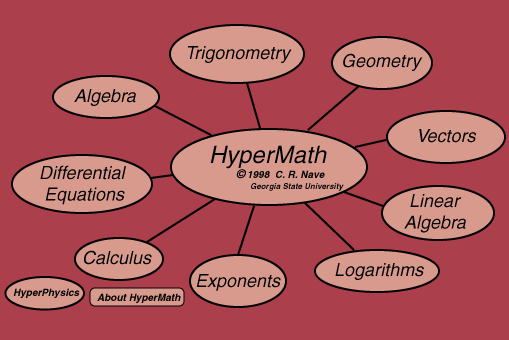Applied statistics concepts
Index

Math ReferencesMathWorld

SOS Math

 HyperPhysics R Nave
Go Back

# About HyperMath: HyperMath is a growing collection of examples of applied mathematics with links to their applications to problems in physics and astronomy. It is not systematic or complete in any sense, but is a collection of foundation mathematics principles and applications which were collected as the need was encountered in developing the HyperPhysics material.

Index

 HyperPhysics****HyperMath R Nave
Go Back